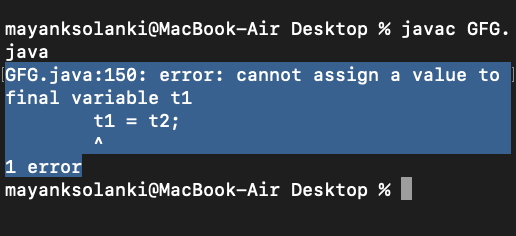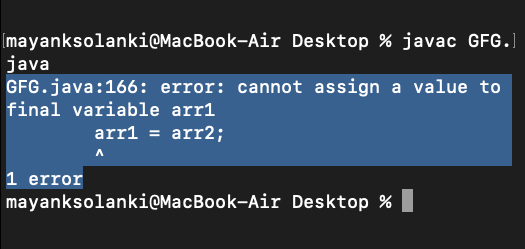# Final Arrays in Java

• Difficulty Level : Easy
• Last Updated : 03 Jun, 2022

As we all know final variable declared can only be initialized once whereas the reference variable once declared final can never be reassigned as it will start referring to another object which makes usage of the final impracticable. But note here that with final we are bound not to refer to another object but within the object data can be changed which means we can change the state of the object but not reference. So, as we all know that arrays are also an object so final arrays come into play. The same concept does apply to final arrays that are we can not make the final array refer to some other array but the data within an array that is made final can be changed/manipulated.

Illustration:

## Java

 `// Java Program to Illustrate Final Arrays``// Can Be Reassigned But Not Re-referred` `import` `java.util.*;` `class` `GFG {``    ``public` `static` `void` `main(String[] args)``    ``{` `        ``final` `int``[] arr = { ``1``, ``2``, ``3``, ``4``, ``5` `};` `        ``arr[``4``] = ``1``;` `        ``for` `(``int` `i = ``0``; i < arr.length; i++) {``            ``System.out.println(arr[i]);``        ``}``    ``}``}`

Output

```1
2
3
4
1```

## Java

 `// Java Program to Illustrate Final Arrays` `// Importing required classes``import` `java.util.*;` `// Main class``class` `GFG {` `    ``// Main driver method``    ``public` `static` `void` `main(String args[])``    ``{` `        ``// Declaring a final array``        ``final` `int` `arr[] = { ``1``, ``2``, ``3``, ``4``, ``5` `};` `        ``// Iterating over integer array``        ``for` `(``int` `i = ``0``; i < arr.length; i++) {``            ``arr[i] = arr[i] * ``10``;``            ``System.out.println(arr[i]);``        ``}``    ``}``}`

Output

```10
20
30
40
50```

Output Explanation: The array arr is declared as final, but the elements of an array are changed without any problem. Arrays are objects and object variables are always references in Java. So, when we declare an object variable as final, it means that the variable cannot be changed to refer to anything else.

Example A:

## Java

 `// Program 1` `// Main class``class` `Test``{``    ``int` `p = ``20``;``    ``public` `static` `void` `main(String args[])``    ``{``       ``final` `Test t = ``new` `Test();      ``       ``t.p = ``30``;``       ``System.out.println(t.p);  ``    ``}   ``}`

Output

`30`

Example B:

## Java

 `// Java Program to Illustrate Final Arrays``// Where Compiler Error Is Thrown` `// Main class``class` `GFG {` `    ``int` `p = ``20``;` `    ``// Main driver method``    ``public` `static` `void` `main(String args[])``    ``{` `        ``// Creating objects of above class``        ``final` `GFG t1 = ``new` `GFG();``        ``GFG t2 = ``new` `GFG();` `        ``// Assigning values into other objects``        ``t1 = t2;` `        ``System.out.println(t1.p);``    ``}``}`

Output: Compiler Error: cannot assign a value to final variable t1Conclusion: Above program compiles without any error and program 2 fails in compilation. Let us discuss why the error occurred.

So a final array means that the array variable which is actually a reference to an object, cannot be changed to refer to anything else, but the members of the array can be modified. Let us propose an example below justifying the same.

Example:

## Java

 `// Java Program to Illustrate Members in Final Array``// Can be Modified` `// Main class``class` `GFG {` `    ``// Main driver method``    ``public` `static` `void` `main(String args[])``    ``{``        ``// Declaring a final array``        ``final` `int` `arr1[] = { ``1``, ``2``, ``3``, ``4``, ``5` `};` `        ``// Declaring normal integer array``        ``int` `arr2[] = { ``10``, ``20``, ``30``, ``40``, ``50` `};` `        ``// Assigning values to each other``        ``arr2 = arr1;``        ``arr1 = arr2;` `        ``// Now iterating over normal integer array``        ``for` `(``int` `i = ``0``; i < arr2.length; i++)` `            ``// Printing the elements of above array``            ``System.out.println(arr2[i]);``    ``}``}`

Output:Please write comments if you find anything incorrect, or you want to share more information about the topic discussed above.

My Personal Notes arrow_drop_up